Free Printable Worksheets for Third Grade

## Algebra: 1 Step Addition & Subtraction Equations (Set 4)Free algebra worksheet – 1 step addition and subtraction equations. – 15 problems per page – solutions included...

## Algebra: 1 Step Addition & Subtraction Equations (Set 3)Intro algebra worksheet: Find the value of x for the problems listed. – 1 step addition and subtraction equations. – with solutions...

## Algebra: 1 Step Addition & Subtraction Equations (Set 2)Algebra introduction worksheet. 1 step addition and subtraction equations. – 15 problems with solutions...

## Algebra: 1 Step Addition & Subtraction Equations (Set 1)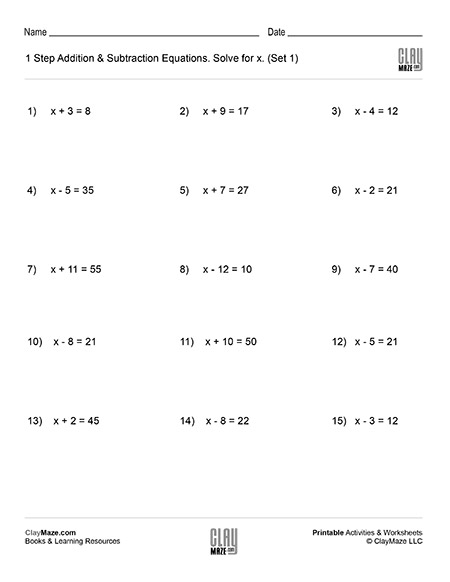This worksheet contains problems that act as an introduction to algebra. The problems are 1 step addition and subtraction equations that ask the student to solve for x. – solutions included...

## 3 digit addition worksheet with regrouping (Set 4)Free math addition worksheet with 3 digit numbers. All problems in this set are on addition with regrouping....

## 3 digit addition worksheet with regrouping (Set 3)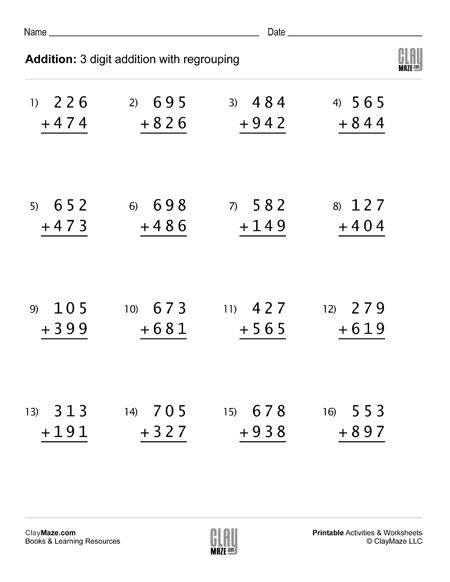This math worksheet contains 16 problems of 3 digit addition with regrouping – good for first grade, second and third grade addition practice....

## 3 digit addition worksheet with regrouping (Set 2)## 3 digit addition worksheet with regrouping (Set 1)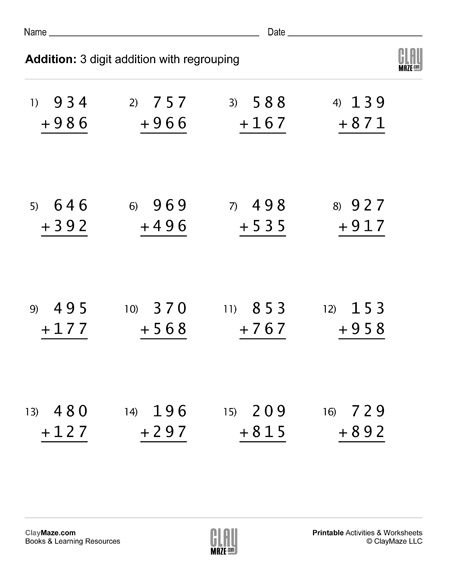## Basic Statistics: Probability I (Set 4)Worksheet on basic probability – The student is asked several questions about the number of shapes in the box and to find the probabilities of selecting different shapes at random....

## Basic Statistics: Probability I (Set 3)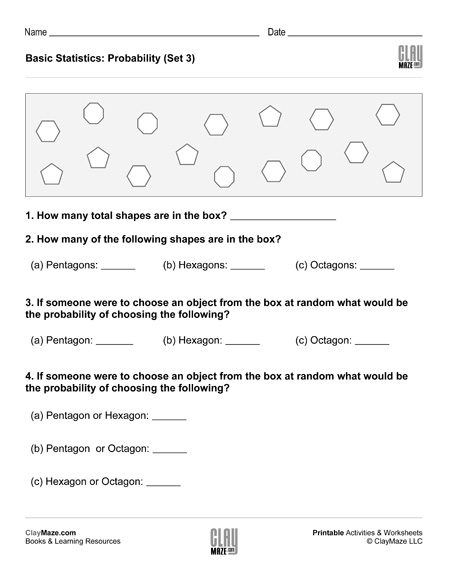This is a basic probability worksheet. The questions are based on the number of shapes in the box and the student is asked to find the probabilities of randomly choosing the different shapes....

## Basic Statistics: Probability I (Set 2)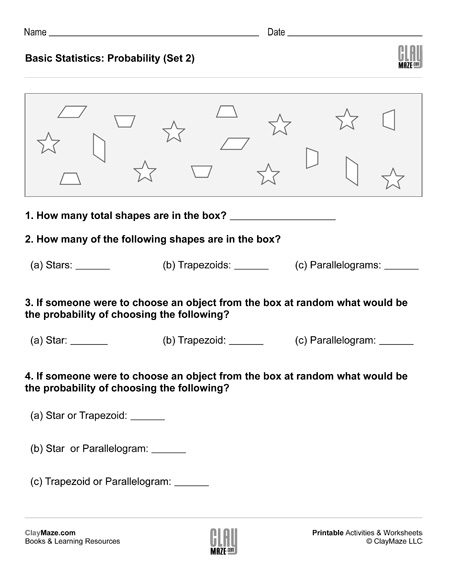This free worksheet covers basic probability. The student is asked several questions about the number of shapes in the box. The student can then use those values to find the probabilities of...

## Basic Statistics: Probability I (Set 1)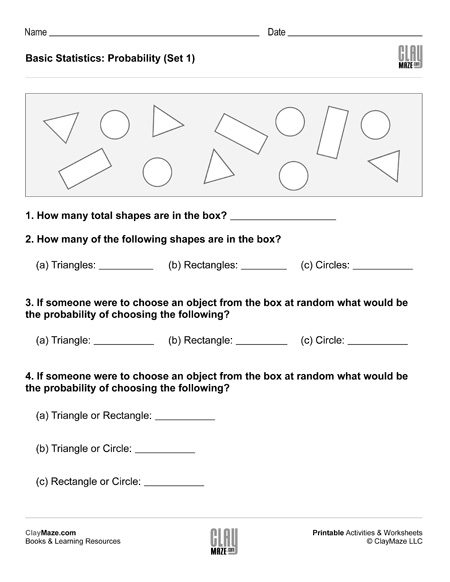This statistics worksheet is on basic probability. There are a number of different shapes in the box and the student is asked to find the probabilities of selecting the different shapes at random....

## Order of Operations I (Set 4)Print this worksheet for practice using the correct order of operations. The problems use a combination of addition, subtraction, multiplication and division....

## Order of Operations I (Set 3)Download this worksheet for students learning to simplify expressions using the correct order of operations. The problems use addition, subtraction, multiplication and division....

## Order of Operations I (Set 2)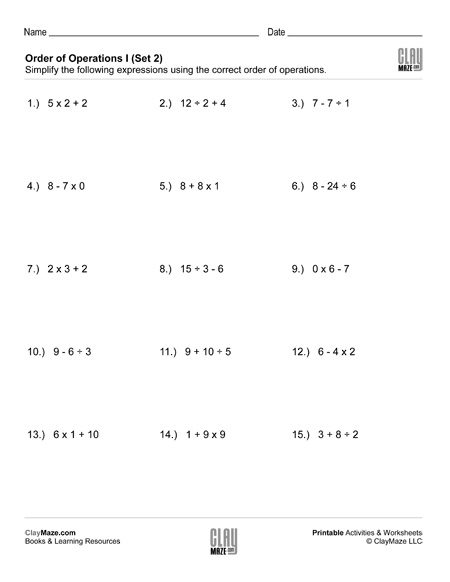This worksheet is great for students learning to use the correct order of operations to simplify expressions.  The problems involve 3 numbers using addition or subtraction combined with...